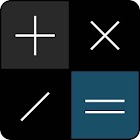• 검색 유형

모든 Android 애플리케이션 카테고리

모든 Android 게임 카테고리Calculator

by: 11 7.8

7.8 사용자
평가 등급

스크린샷

설명

Calculator app is a free calculator tool that allows users to do multi operations in single line. Just write all the operations on screen and the app calculates the solution with respect to mathematical operators priority rules.

Calculator app is a combination of scientific calculator and simple 4 function calculator, Users can switch between simple calculator mode to scientific calculator mode easily.

Main Properties:

- Main operations : addition, subtraction, multiplication, division
- Scientific operations: trigonometric, logaritmic, exponential
- Swipe down on the screen for calculations history
- Swipe up to on the keyboard for scientific operations on simple mode
- Full screen keypad on scientific mode

: 11 리뷰

"우수"

7.8